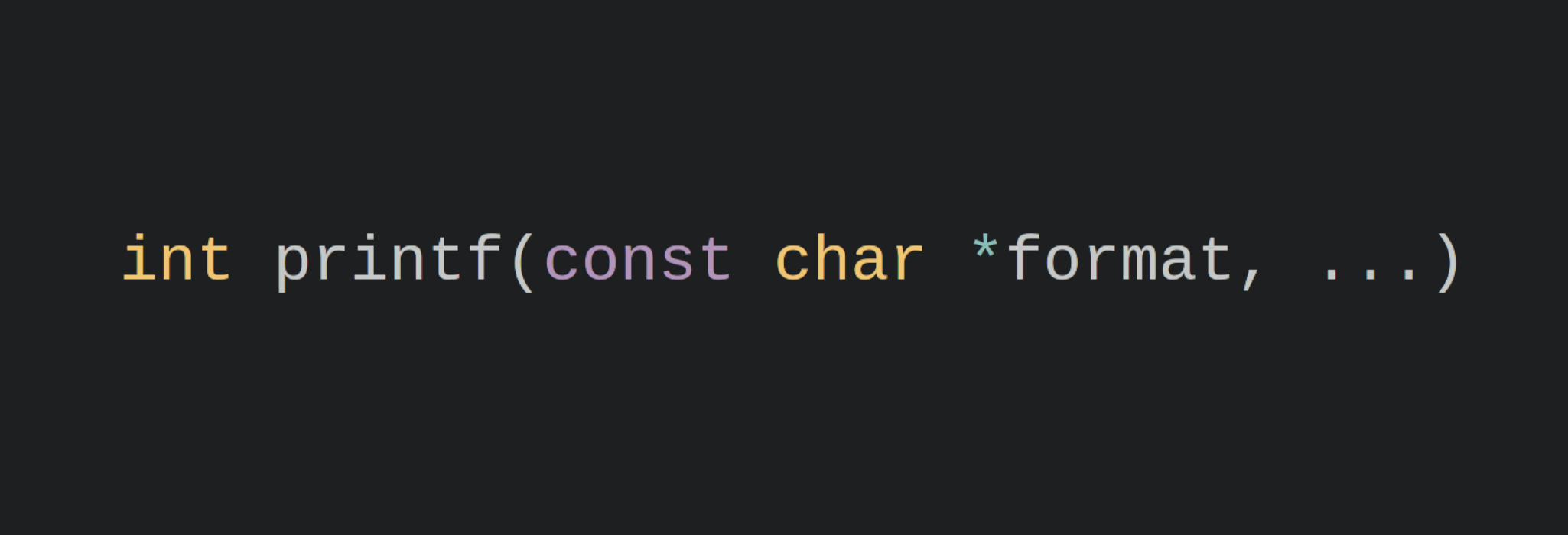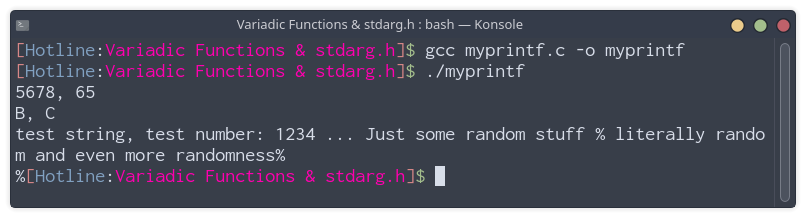# printf

If you’ve ever programmed using C or C++, you’ve probably come across the function printf(). It’s a very popular function and all of C/C++ coders have seen it before.

printf (short for print formatted) basically prints formatted data to standard output (STDOUT). The function does so by using its format parameter, and the variables you want to print.

int printf(const char *format, ...)


printf’s function declaration.

## Format Specifiers

const char *format is the format string, it can contain pretty much any text you want, you just need to include a format specifier (which starts with %) for each variable you will pass to printf depending on how you want to represent the data.

Format Specifier Data Type
%s String (char *)
%d Signed decimal Integer (base 10)
%x Unsigned hexadecimal integer (base 16)
%c Character
%f Floating point integer

For example, if you want to print an integer variable containing a sum of numbers using printf, you can do the following: printf("The sum is: %d", sum).

If you want to know about other format specifiers, check them out here.

You can also include multiple variables in the same printf call. For example:

printf("I live in %d, %s", buildingNumber, streetName);

char c = 'A';
printf("Character %c has an ASCII value of %d, which is %x in hex", c, c, c);


If you want to know more about how printf works on a deeper level, MaiZure has written an amazing blog post on Tearing apart printf, go ahead and give it a read!

Now enough talking about printf itself, let’s get to our main topic. Functions like printf in mathematics and computer science are called variadic functions. They are functions which can accept a variable number of arguments. i.e. they don’t have the same number of arguments every time they’re called. A function like sqrt() will always have exactly one parameter, while a function like printf can’t have a fixed number of arguments because its number of arguments depends on the format string the user decided to pass to the function. A lot of programming languages support variadic functions. However, let’s stick to C since we were talking about printf.

# stdarg.h

In C, you can include the stdarg.h header to declare variadic functions. To specify that a function will have variable arguments, you add the ... argument with no data type next to it.

Example: int addNumbers(int n, ...);

stdarg.h declares the va_list type which stores the the passed parameters to the variadic functions. It also includes a couple of macros to help you work with va_list, we’ll take a look at three of them only:

• va_start(va_list, lastNamedMember) which initialises the va_list object and requires the last named member in the function’s arguments.
• i.e. if the function signature is void func(int a, char b, int c, ...) the last named member is c, as the ... is unnamed.
• va_arg(va_list, type) which returns the next parameter as the specified type.
• va_end(va_list) which cleans up the va_list object the user previously initialised.

The following is an example of the previously mentioned addNumbers(int n, ...) function. While this is an overly complicated and meaningless usage of variadic functions, it is just an example.

#include <stdio.h>
#include <stdarg.h>

{
//Initialise va_list
va_list parameters;
va_start(parameters, n);

int sum = 0;

//Retrieve each argument and add it to sum
while (n--)
{
sum += va_arg(parameters, int);
}

//Clean up
va_end(parameters);

return sum;
}

int main()
{
int sum = addNumbers(3, 1, 2, 3);
printf("The sum is: %d\n", sum); //Will print 6

return 0;
}


## myprintf example

Now, let’s rewrite a small version of printf which supports strings (%s), characters (%c), and decimal numbers (%d). We will only focus on these data representations and also handle escaping % signs when calling printf by adding another percent sign: %%.

#include <stdio.h>
#include <string.h>
#include <stdarg.h>

int myprintf(const char* format, ...)
{
//Initialise the va_list
va_list parameters;
va_start(parameters, format);

int len = strlen(format);
for (int i = 0; i < len; i++)
{
// Check that it isn't the second % in %%
if (format[i] == '%' && i != len-1)
{
// Char, just print it
if (format[i+1] == 'c')
{
//For some reason char has to be int int va_arg
char argument = va_arg(parameters, int);
putchar(argument);
//Increment i to skip the second character of the format specifier
i++;
}
// String, print every character until a '\0' is found
else if (format[i+1]  == 's')
{
char *argument = (char *) va_arg(parameters, char *);
while (*argument != '\0')
{
putchar(*argument);
argument++;
}
i++;
}
// Decimal integer, convert to string using itoa() and then print
else if (format[i+1] == 'd')
{
int argument = va_arg(parameters, int);
char integerString;
itoa(argument, integerString, 10);

int len = strlen(integerString);

for (int j = 0; j < len; j++)
putchar(integerString[j]);

i++;
}
// Escaped % sign, print it
else if (format[i+1] == '%')
{
putchar('%');
i++;
}
}
else //Print the character if it isn't a format specifier or an escaped %
{
putchar(format[i]);
}
}

// Clean up
va_end(parameters);
}

int main()
{
myprintf("%d, %d\n", 5678, 'A');
myprintf("%c, %c\n", 'B', 67);
myprintf("%s, test number: %d ... Just some random stuff %% %s %c%c%c even more randomness%% \n%%", "test string", 1234, "literally random", 'a', 'n', 'd');
return 0;
}itoa() isn’t an ANSI C function, I just used the implementation provided here because converting an int to a string isn’t the focus of this post.

Thanks a lot for reading! I hope you learned something from this post or at least found it interesting.

2021 has been a very busy year for me and I haven’t had the time to properly write anything in quite some time so I am glad I was able to write this one, I hope you enjoyed reading it as much as I enjoyed writing it! Share it with any of your friends who may find it interesting.